Ask your WordPress questions! Pay money and get answers fast!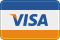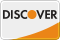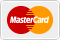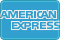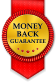# Gravity Form Error with Arrays after 9th counter WordPress

• REFUNDED

I have the following two custom functions, one for pre-rendering a form based on a post's custom taxonomy and the other to update it after submission. The field is a checkbox. The field fully pre-renders all of the possible terms for the custom taxonomy, but the isSelect will only work for the first 9 fields. The same goes for after submission -- it will only update the post for the first 9 possible terms then it omits them.

Here is the code for pre render:
``` add_filter('gform_pre_render_2', 'GF_prepopulate_cat'); add_filter('gform_admin_pre_render_2', 'GF_prepopulate_cat'); function GF_prepopulate_cat(\$form){ foreach(\$form["fields"] as &\$field){ if ( \$field["id"] == 100 ){ \$parent = '26'; \$cats = get_terms('portfolio-item-category', array( 'hide_empty' => false, 'parent' => \$parent )); \$field['choices'] = array(); foreach (\$cats as \$cat){ \$name = \$cat->name; \$val = \$cat->term_id; if ( isset( \$_GET["gform_post_id"] ) ) { \$ID = \$_GET["gform_post_id"]; \$term_list = wp_get_post_terms( \$ID, 'portfolio-item-category' , array( "fields" => "ids" ) ); if (in_array(\$val, \$term_list)) { \$select = true;} else { \$select = false; } }else{ \$select = false; } \$field['choices'][] = array( 'text' => \$name, 'value' => \$val, 'isSelected' => \$select); } } } return \$form; }```

Here is after submission:

``` add_action("gform_after_submission_2", "GF_update_post_2", 10, 2); function GF_update_post_2 (\$entry, \$form) { global \$wpdb, \$post; \$ID = \$entry["post_id"]; if (!isset(\$_GET["gform_post_id"])){ foreach(\$form["fields"] as \$field){ ob_start(); if( \$field["id"] == 100 ){ \$all_terms = \$field['choices']; for(\$i = 1; \$i <= count(\$all_terms); \$i++){ \$id = \$field['id']; \$submitted_term = \$entry["{\$field['id']}.\$i"]; if (!empty(\$submitted_term)){ echo \$submitted_term.' ,'; } } } } \$output_string = ob_get_contents(); ob_end_clean(); wp_set_post_terms(\$ID,\$output_string, 'portfolio-item-category' ); }}```

2013-08-23

Hello Kyle

I would like to view the actual things going on.
If possible can I connect you through team viewer and sort this out.

I will PM my skype ID

If possible Can you post the screenshot of your form .It will be useful to understand the concept best.

Here is the image [[LINK href="http://imgur.com/SVrN9ld"]]link[[/LINK]] You can see that the first 9 pre render, and they also will actively update after submission. However, none of the inputs work.

```<?php add_filter('gform_pre_render_2', 'GF_prepopulate_cat'); add_filter('gform_admin_pre_render_2', 'GF_prepopulate_cat'); function GF_prepopulate_cat(\$form){ foreach(\$form["fields"] as &\$field){ if ( \$field["id"] == 100 ){ \$parent = '26'; \$cats = get_terms('portfolio-item-category', array( 'hide_empty' => false, 'parent' => \$parent )); \$field['choices'] = array(); foreach (\$cats as \$cat){ \$name = \$cat->name; \$val = \$cat->term_id; if ( isset( \$_GET["gform_post_id"] ) ) { \$ID = \$_GET["gform_post_id"]; \$term_list = wp_get_post_terms( \$ID, 'portfolio-item-category' , array( "fields" => "ids" ) ); if (in_array(\$val, \$term_list)) { \$field['choices'][] = array( 'text' => \$name, 'value' => \$val, 'isSelected' => true); } else { \$field['choices'][] = array( 'text' => \$name, 'value' => \$val, 'isSelected' => false ); } }else{ \$field['choices'][] = array( 'text' => \$name, 'value' => \$val, 'isSelected' => false); } } } } return \$form; }```